# Selection

Next Tuesday you will fill out teacher evaluations.

Next week will be another good week. Tuesday we will learn to use ModelDB and Thursday we will do abrieviated Projects.

First, the homework from Lab W.

## What is Model Selection?

What is model selection? To answer this question let's contrast model selection with parameter estimation. In the Optimization Lab, we fit lines to data. We were doing parameter estimation, trying to estimate the parameters m and b, in the formula for a line y = m*x + b. Recall my examples: we fit a line to nearly linear data and another line to nearly parabolic data.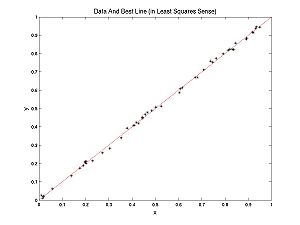Fitting a line to nearly linear data. Click for full size image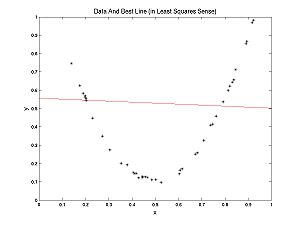Fitting a line to nearly parabolic data. Click for full size image

Clearly, in the second case, it would be more appropriate to fit a parabola to the data, rather than a line. How would we do that?

A parabola is described by the formula: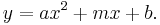$y = a x^2 + m x + b.$ This is a line with a new term,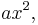$a x^2,$ added. To fit a parabola the procedure is almost the same. First determine the errors in the data: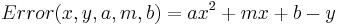$Error(x,y,a,m,b) = a x^2 + m x + b - y$

Now define the penalties, (let's be sensible and use LS rather the UG penalties):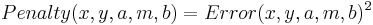$Penalty(x,y,a,m,b) = Error(x,y,a,m,b)^2$

And finally the cost function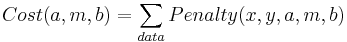$Cost(a,m,b) = \sum_{data} Penalty(x,y,a,m,b)$

Now we tweak the three parameters (a,m,b) using, for example, the Nelder-Meade method we studied last week. Of course MATLAB has its own routines for solving this problem based on Linear Algebra that are much better than Nelder-Meade.

What if we fit a parabola to the nearly linear data? Note that if we set a = 0, we get y = m*x + b again. Thus a line is a parabola. But there was some noise in the data, so if you are allowed to tweak the parameter a as well as m and b, you can expect a better (lower cost) fit (you are guaranteed that the fit is no worse). So why should we bother fitting lines to data? Why not just always fit parabolas?

But wait, why stop at parabolas? An nth degree polynomial has the form: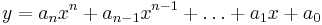$y = a_n x^n + a_{n-1} x^{n-1} + \dots + a_1 x + a_0$

For example, a 0th degree polynomial is a constant function, 1st degree is a line, 2nd degree is parabola, etc. If we have m data points a unique polynomial of degree m-1 will fit exactly (provided the x-values of the data points are not repeated). Some (no longer unique) higher degree polynomials will also fit exactly under the same stipulation.

So why don't we fit with a high enough polynomial so that there is no error? If we have 100 data points why not use a 99 degree polynomial? Then there is no errors, no penalties, and zero cost! Perfect fit!?

There are two reasons you don't want to do that:

• Philosophical: parsimony. The model that generated our data (in both examples above) is a low degree polynomial plus noise. This model can be described with only a few parameters (a,m,b) and NoiseLevel. If our data is generated in the lab, we may never know what the exact model is that created the data. But Occam's razor (a standard paradigm in science) tells us we should pick the simplest model that is consistent with the data. The complexity of a model can be quantified with number of parameters. A degree m polynomial has m+1 parameters. Thus a low degree polynomial is preferred unless the data compel the use of a more complicated model.
• Practical: overfitting (subject of next section). Fitting models with too many parameters leads to fits that are tuned in a sensitive way (overfitted) to the particular noise that appeared in the data set used for the fitting. If you run the experiment again, you get different noise. Typically, an overfitted model does a much worse job of predicting the new experiment (with new noise) than a simpler more parsimonious model.

Model selection helps you decide how complicated a model you need to fit the data in a way that is robust to repeating the experiment. What we are going to do today is generate some data (polynomial plus noise) then use Model Selection to decide what degree fits the data best.

For neural models, you might use model selection to decide how many currents, and what types of currents are present in a cell, or how many compartments are needed in a multi-compartment model to reproduce the data.

## An Example Of Overfitting

I generated some data from a noisy parabola. The noise level was 0.1 which is fairly large.

Here is the best fitting constant function: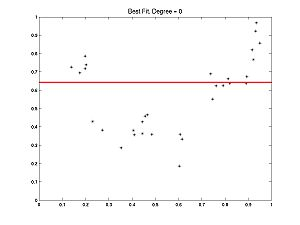Fitting to noisy parabolic data. Click for full size image

Line: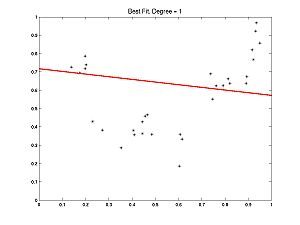Fitting to noisy parabolic data. Click for full size image

Parabola: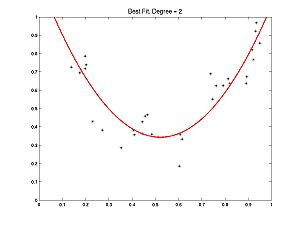Fitting to noisy parabolic data. Click for full size image

We used a similar (but undoubtedly not exactly the same) parabola to generate the data.

From here on, we start to have too many parameters:

Degree 3: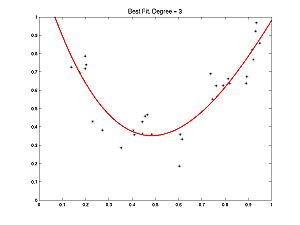Fitting to noisy parabolic data. Click for full size image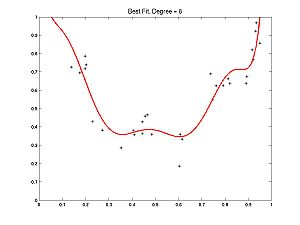Fitting to noisy parabolic data. Click for full size image

This one is starting to look a little weird.

Let's try Degree 20:Fitting to noisy parabolic data. Click for full size image

This goes through all the points OK, but it oscillates where there are spaces. Notice how much it oscillates at the end, it goes completely in the wrong direction! Now let's use the 20 degree polynomial to predict how well the model behaves on a new experiment with a new random seed. Here is the result:

Notice that data points got stuck in the spaces where there were oscillations in the prediction and were poorly modelled by the high degree polynomial. This is overfitting.

## Model Selection Statistics

We will use two satistics:

There are more.

The formula for these statistics are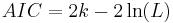$AIC = 2 k - 2 \ln(L)$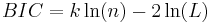$BIC = k \ln(n) - 2 \ln (L)$

where

• k is the number of parameters (degree of polynomial plus 1)
• L is the maximum value of the likelihood (related to the minimizing LS-Cost by a simple formula: likelihood = "a negative constant"*LS-cost)
• n is the number of data points (used for BIC but not for AIC)

The model with the lowest AIC or BIC wins. Note that the statistics is a simple tradeoff between fewer parameters and greater likelihood.

If you are comparing an infinite family of models, such as polynomials of all degrees, you need a model selection strategy. This is the one I used:

• Increase degree, recalculate statistic. If statistic is lower (better model) keep going.
• Repeat previous step until you get a higher statistic (worse model), at which point you stop and the best model so far (second to last) wins.

Following this steps you don't need to try infinitely many degrees.

Here is the result of model selection with the overfitting example, using the AIC

>> AICselect(pdata)
Degree: 0 AIC: 150.9056: BASELINE
Degree: 1 AIC: 145.8956: BETTER
Degree: 2 AIC: -60.7332: BETTER
Degree: 3 AIC: -61.2846: BETTER
Degree: 4 AIC: -59.2946: WORSE
AIC deems the answer DEGREE 3
RECALL: The polynomial that generated the data had DEGREE 2


Notice it overestimates the degree. The BIC generally penalizes extra parameters more and gives a lower estimate of degree. Here is a run of BIC on the same data set:

>> BICselect(pdata)
Degree: 0 BIC: 152.4609: BASELINE
Degree: 1 BIC: 149.0063: BETTER
Degree: 2 BIC: -56.0672: BETTER
Degree: 3 BIC: -55.0632: WORSE
BIC deems the answer DEGREE 2
RECALL: The polynomial that generated the data had DEGREE 2


## Try it Yourself

Next generate some data. Use the GenPolyData.m file that was introduced in the previous lecture. Remember, here are the steps:

• In MATLAB, with the above functions in your path, type the following command, causing MATLAB to open a figure then hang:
pdata = GenPolyData;

• Move mouse into the axes of the figure and press left mouse button.
• Repeat (move mouse and press left mouse button) until satisfied. Each press increases the degree of the polynomial generating data by one and the polynomial goes through the selected point.
• When the desired polynomial is constructed, move mouse outside of axes, but still inside figure, and press mouse button.
• Now at the command prompt, enter noise level, number of data points, and random seed. If you entered something into the keyboard while you were selecting the polynomial, it will cause problems at this step. If this happens start over and be more careful.

GenPolyData returns the structure "pdata" with your data set and meta-data about how it was generated. Now let's try some different degree polynomial fits.

• Type into the MATLAB prompt
PolyFit_Inspector(pdata)

• Follow the prompt for degree of polynomial to fit to your data. Type whatever degree you want within bounds (but note that if the degree is too high the problem is hard computationally and prone to numerical errors -- fits might be bad). I tried 3:
>> PolyFit_Inspector(pdata)
Degree to fit (any integer between 0 and 34) or -1 to quit: 3
Degree:
Sum of Penalties: 0.27571
Log-Likelihood (with NoiseLevel known): 34.6423
Akaike Information Criterion: -61.2846
Bayesian Information Criterion: -55.0632
New random seed for Cross-Validation (0 original), or -1 for new degree:

• It printed the information above and prompted you again. Also check the figure, it should have plotted the best fitting degree 3 polynomial to the data:Fitting to noisy parabolic data. Click for full size image
• The prompt is for a new seed to generate new (cross-validation) data. If you want to start over with a new degree type in -1.
• Repeat with new seeds, then type in -1 and repeat with new degrees. Have fun.
• When you are ready to run AIC selection strategy, type
AICselect(pdata)

• And BIC
BICselect(pdata)

• When you want a new data set, start over with
pdata2 = GenPolyData;


Here I have used a different name (pdata2 instead of pdata) for the structure to save the old data set. It will only overwrite the data set if you give it the same name. For the homework, which requires you to work with many datasets, might help you to give them more intuitive names:

LinearData_HiNoise_30pnts = GenPolyData;


You can also rename data sets (i.e. copy pdata after it has been defined by GenPolyData and possibly used by the other functions):

LinearData_HiNoise_30pnts = pdata;


## Homework

Homework X.1 For our class of problems when does model selection work well, and when does it work poorly? Try different combinations of low/high levels of noise, and lots of/few data points. For this part, use a fairly simple polynomial to generate the data. Note: I don't know what will happen if you try to fit polynomial with degree higher than the number of data points. Maybe an error.

Homework X.2 Investigate the effects of the polynomial's shape on model selection. Use both high and low degree polynomials for this problem. For low degree polynomials, try, for example, a parabola that is almost a line over the region of the data: (0,1). Get creative!